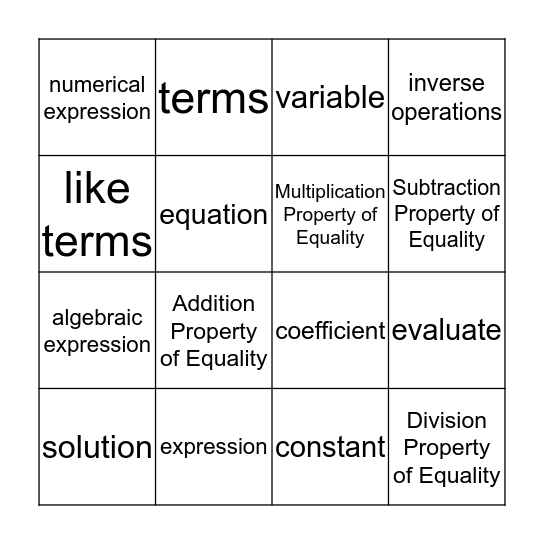# Math Bingo-like GameThis bingo card has 16 words: expression, evaluate, variable, numerical expression, algebraic expression, terms, coefficient, constant, like terms, equation, solution, inverse operations, Addition Property of Equality, Subtraction Property of Equality, Multiplication Property of Equality and Division Property of Equality.

## Play Online